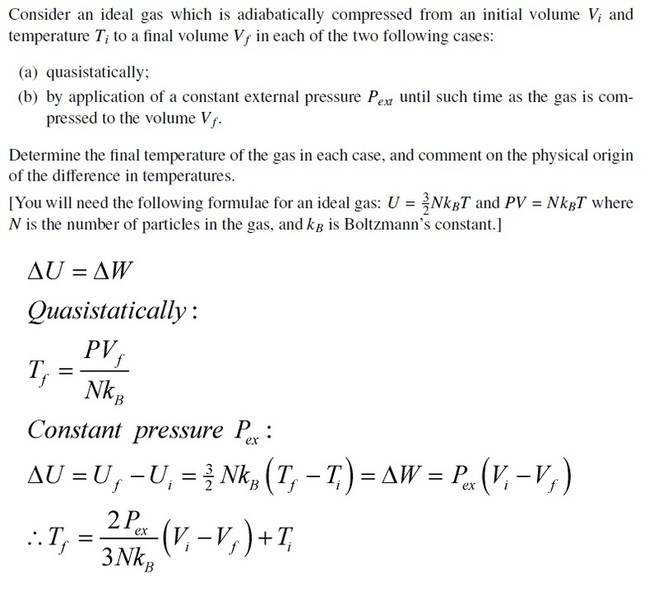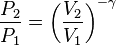# Thermo question, two methods of changing volume/temp

Gregerdoes this look right?

for the quasistatic case you can use thermodynamics to find the temperature at any time,

for the other case you have to use dU=dW and so on since its not quasistatic

Homework Helperdoes this look right?

for the quasistatic case you can use thermodynamics to find the temperature at any time,

for the other case you have to use dU=dW and so on since its not quasistatic

It is correct for the constant external pressure. But what is the final temperature in the quasi-static case? It is true that Tf=PVf/(NKB) but P changes during the process. How do you get the final pressure Pf?

ehild

Greger
Oh right,

so the quasistatic case should be:

Tf=PfVf/(NKB)

for some reason i forgot that the pressure was changing in that case as well,

this makes more sense, in the quasistatic case you can find the state of the system at any point in terms of the state variables, and P is one of those too!

thanks ehild =]

Homework Helper
Can you show what you got? Just to complete the solution, so as other people learn from it.ehild

Greger
quasistatic case :

Tf=PfVf/(NKB)

under constant pressue:

Tf=2Pex(Vi-Vf)/3NkB + Ti

Homework Helper
quasistatic case :

Tf=PfVf/(NKB)

under constant pressue:

Tf=2Pex(Vi-Vf)/3NkB + Ti

Well, it is not the solution yet. You need to give Tf in terms of the initial and final volumes and the initial temperature. Do you know the equation that governs a quasistatic adiabatic process?

ehild

Greger
Oh,

do you mean something likewhere gamma is the ratio of heat capacities under constant pressure / volume?

Homework Helper
Yes. But you can combine it with the ideal gas law to give an equation between V and T.

ehild

Greger
yea there's afew like

TV^(gamma - 1)=constant

PV^gamma = const

but wouldn't mucking around with these and introducing them into my problem make it like

Tf=PfVf/(NKB)=PiVf1-λViλ/(NKB)

using λ as gamma

PV^gamma = const = C say

Tf=CVf1-λ/NKB

then i have this unknown constant C

Homework Helper
yea there's afew like

TV^(gamma - 1)=constant

That is a good start. You can find that constant as you know the initial volume and temperature:
TfVfγ-1=TiViγ-1.
From the equation for the internal energy you can see that it is a mono-atomic gas, so you know Cv, and you also know the relation between Cv and Cp. What is γ-1 then?

ehild

Greger
C=NKb Tf Vf^(γ-1)=NKb TiVi^(γ-1)

Tf= Ti(Vf Vi)^(γ-1)

for γ=Cp/Cv

Tf= Ti(Vf Vi)^(Cp/Cv-1)

is that kind of what you mean?

Homework Helper
C=NKb Tf Vf^(γ-1)=NKb TiVi^(γ-1)

Tf= Ti(Vf Vi)^(γ-1)

for γ=Cp/Cv

Tf= Ti(Vf Vi)^(Cp/Cv-1)

is that kind of what you mean?

Yes, but you made a little error:

Tf=Ti(Vi/Vf)γ-1.
And you know the numerical value of Cp/Cv what is it?

ehild

Greger
oh whoops,

Tf=Ti(Vi/Vf)5/3-1.

Tf=Ti(Vi/Vf)2/3.

thanks ehild

Homework Helper
It is the SOLUTION nowehild